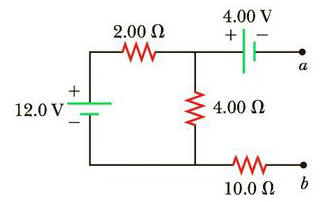Chapter 18, Problem 47AP

Chapter
Section
Textbook Problem

(a) Calculate the potential difference between points a and b in Figure P18.47 and (b) identify which point is at the higher potential.Figure P18.47

(a)

To determine
The potential difference between the points a and b.

Explanation

No current flows through the 4.00 V battery and 10.0Ω resistor. This is because the circuit is open at points a and b.

Formula to calculate the current through the closed loop is,

I=ΔVReq

• Req is the equivalent resistance of the loop.
• ΔV is the potential difference.

The equivalent resistance is,

Req=(2.00Ω)+(4.00Ω)=6.00Ω

• ε0 is the permittivity of free space.
• A is the cross sectional area.

Substitute 6.00Ω for R and 12.0 V for ΔV

(b)

To determine
The point with higher potential.

Still sussing out bartleby?

Check out a sample textbook solution.

See a sample solution

The Solution to Your Study Problems

Bartleby provides explanations to thousands of textbook problems written by our experts, many with advanced degrees!

Get Started